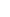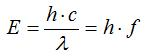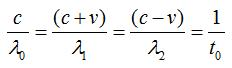27. ENERGY OF A PHOTONId like to touch upon this topic now that it came up. In the figure below, the duration of the emission of signals and the duration of the receiving of signals at arrival targets are shown in the same figure based on wavelengths of the electromagnetic waves.Division of signal wavelength by signal speed gives us the duration. Wavelength / Signal Speed = Duration of emission or receiving of wavelengthSince signal speed may vary relative to the reference systems that send or receive signals, we calculate this duration in two ways. 1. Duration of emission of the wavelength relative to the reference system of the transmitter (OUTGOING signals) 2. Duration of receiving of the wavelength relative to the reference system of the arrival target (INCOMING signals).  These durations are already shown in the figure.Energy of a Photon In physics, energy of a photon is represented by the equationE: energy of the photon, h: Planck's constant, c: speed of light, λ: wavelength of the photon, f: frequency of the wave (photon)Lets look at the figure now. Even though the lengths of the wavelengths that set out from the transmitter are different, we know that they emit in the same durations. Nevertheless, it is different for the receiving side. The fact that the speed of the INCOMING signal is always c relative to the arrival target in the interactions between objects that are in motion increases or decreases the duration of receiving depending on the length of the wavelength. The difference between the durations of emission and receiving of the wavelength is perceived as an increase or decrease in the energy of the electromagnetic wave (photon). Let's look at the side where the signal transmitter is. Electromagnetic waves that are emitted over λ0, λ1, λ2 wavelengths, in reality, carry an equal amount of energy because they are emitted from the same source with the same frequency value. We can see this easily in the equations below.By making use of the duration of emission of wavelengths, we can write the following equation.We multiply the sides of equation  with Planck's constant. In this way, we get the energies of the waves that are emitted.The equation that shows energy of a photon:We reach the following equation  from  and . As can be seen, energies of the waves that are emitted relative to the receiver side are equal.However, as the duration of receiving of signal wavelengths at arrival targets are different, this energy is perceived as if it has a different energy.The energies of the coming waves relative to the receiver side:As can be seen, we can express a photons energy based on the duration of its emission or receiving.E: energy of the photon h: Planck's constant t: duration of emission/receiving of the photonThe equation (E=h/t) shows an interesting point, as well. A sentence like this comes to the mind of a person willy-nilly: Energies of all photons are actually equal. The amount of this energy is equal to Planck's constant; the thing that creates the difference is in how much time is the energy is received or emitted.Naturally, no matter at which energy level it is emitted, the effect of the photon comes out at its arrival target.  Does the energy of a photon perceived at its arrival target represent the real energy of the photon? As can be seen, we can give the answer No to this question.  Is the real energy of a photon the energy at the moment that it sets out from the source? I will prefer to leave this question unanswered.If we think that, logically, the t value in the equationchanges in 0-1 interval, the smaller we make the t value, the more we will compress an energy that is equal to Planck's constant and, as a result of this, the more will be the effect that the photon creates. It is a bit creepy equation. When the features of (c-v) in (c+v) (c-v) mathematics is taken into consideration, the fact that this compression can reach an unbelievable extent and go up to incredible energy levels. Who knows, maybe nature has a precaution for such dangerous situations.If you are thinking about traveling at the speed of light and if you set out without paying attention to this equation, I can say that you will evaporate in a very short time.